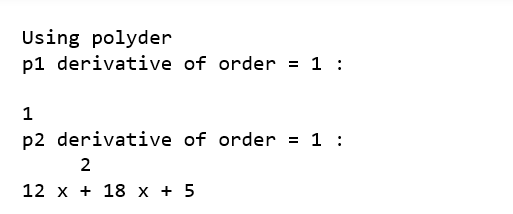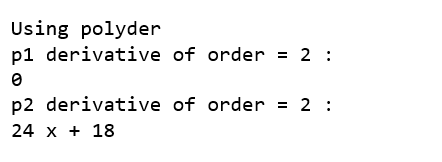numpy.polyder() in Python

• Last Updated : 04 Dec, 2020

The numpy.polyder() method evaluates the derivative of a polynomial with specified order.

Syntax :numpy.polyder(p, m)
Parameters :
p : [array_like or poly1D]the polynomial coefficients are given in decreasing order of powers. If the second parameter (root) is set to True then array values are the roots of the polynomial equation.
For example : poly1d(3, 2, 6) = 3x2 + 2x + 6

m : [int, optional] Order of differentiation.

Return: Derivative of polynomial.

Code : Python code explaining polyder()

 # Python code explaining # numpy.polyder()    # importing librariesimport numpy as npimport pandas as pd  # Constructing polynomial p1 = np.poly1d([1, 2]) p2 = np.poly1d([4, 9, 5, 4])     print ("P1 : ", p1) print ("\n p2 : \n", p2)# Solve for x = 2 print ("\n\np1 at x = 2 : ", p1(2)) print ("p2 at x = 2 : ", p2(2))a = np.polyder(p1, 1)b = np.polyder(p2, 1)print ("\n\nUsing polyder")print ("p1 derivative of order = 1 : \n", a) print ("p2 derivative of order = 1 : \n", b)a = np.polyder(p1, 2)b = np.polyder(p2, 2)print ("\n\nUsing polyder")print ("p1 derivative of order = 2 : ", a) print ("p2 derivative of order = 2 : ", b)My Personal Notes arrow_drop_up